# Star Delta Transformation

## Star Delta Transformation – how it works

The star-delta conversion is a tool for simplifying complex resistor networks. Here, the three resistors are reconnected from delta to star - and vice versa, with correspondingly altered resistance values, so that the ratios between the pins remain the same in both circuit variants.

### Delta to Star Transformation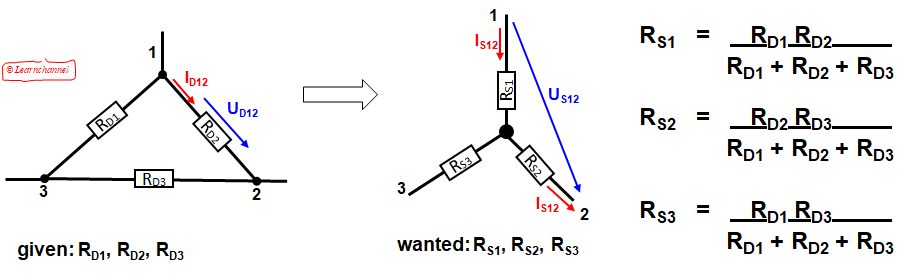Transformation Delta to Star explained

We proof this:

Condition for the calculation of the resistors RS1, RS2, RS3: Current and voltage values must be the same between the terminals in both circuit types!

We consider the pins 1 and 2:

UD12  =  US12    =>   resulting in RD12 = RS12
ID12        IS12

We consider the pins 2 und 3:

UD23  =  US23    =>   resulting in RD23 = RS23
ID23        IS23

We consider the pins 1 und 3:

UD13  =  US13    =>   resulting in RD13 = RS13
ID13        IS13

Calculation of the resistances between the pins:

RD12 = RD2 ΙΙ (RD1 + RD3)  = RD2 (RD1 + RD3)    must be equal to  RS12 = RS1 + RS2
RD1 + RD2 + RD3

Resulting in:

RD12RD1RD2 + RD2RD3  = RS12 = RS1 + RS2                   …Eq.(1)
.           RD1 + RD2 + RD3

also:

RD31 = RD1RD2 + RD1RD3    =  RS31 = RS3 + RS1           …Eq.(2)
.         RD1 + RD2 + RD3

RD23 = RD3RD1 + RD3RD2   =  RS23 = RS2 + RS3            …Eq.(3)
.         RD1 + RD2 + RD3

We add Eq.(1) + Eq.(2) - Eq.(3):

RD1RD2 + RD2RD3 + RD1RD2 + RD1RD3 - (RD3RD1 + RD3RD2)  = RS1 + RS2 + RS1 + RS3 - RS2 - RS3   = 2RS1                                                                RD1 + RD2 + RD3

We get as a result:

RS1     RD1 RD2    .
.         RD1 + RD2 + RD3

The same way we get:

RS2     RD2 RD3    .
.         RD1 + RD2 + RD3

RS3     RD1 RD3          .
.         RD1 + RD2 + RD3

If the delta connected system has the same resistance RD at its three sides then the equivalent star resistance RS will be:

RS     RD RD        . = RD  .
.         RD + RD + RD      3

### Star to Delta Transformation

We proceed in the same way for the transformation from star to triangle. The resistors RS1, RS2 and RS3 of the star circuit are converted into the resistors RD1, RD2 and RD3 of the delta circuit with the corresponding resistance values in such a way that the current and voltage values between terminals 1 to 3 are identical.Star to Delta Transformation

We proof this:
In the star connection, the equivalent resistance between the connection points 1 and 2 (resp.3) is obtained by short-circuiting the connections 2 and 3. Thus, an equivalent conductance is formed from RS1 and the parallel connection of RS2 with RS3.
If the same points are short-circuited in the equivalent delta circuit, the total conductance results from the parallel connection of the resistors RD1 and RD2.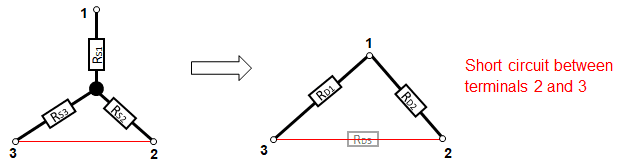Star to Delta Transformation - how it works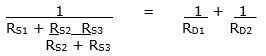Left side results in a common denominator: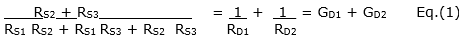The same applied to terminal 3 and short-circuited terminals 1 and 2: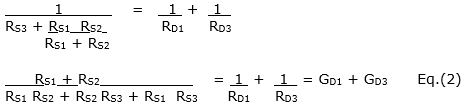The same applied to terminal 2 and short-circuited terminals 1 and 3: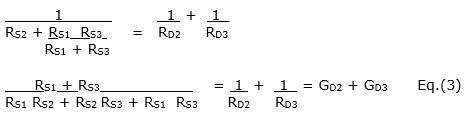We add:   Eq. (1) + Eq. (2)  - Eq. (3):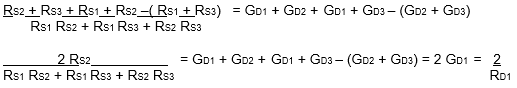Solve this equation for RD1: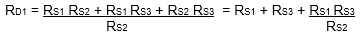The same procedure applies to the remaining branches or resistors.